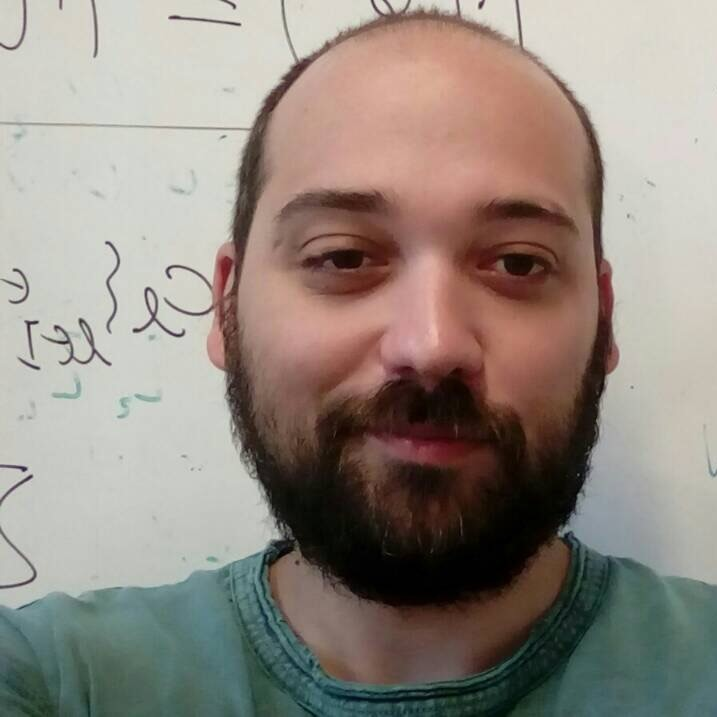People

# Dr Georgios Papamikos

Lecturer
School of Mathematics, Statistics and Actuarial Science (SMSAS)• ### Telephone

+44 (0) 1206 874931

• ### Location

2.529, Colchester Campus

## Research and professional activities

### Research interests

#### Continuous and Discrete Integrable Systems

Open to supervise

#### Mathematical Physics

Open to supervise

#### Algebraic methods in the study of Differential Equations

Using the theory of Lie groups and Lie algebras one can study symmetry properties of differential equations (both ordinary and partial). These properties are key ingredient for a deeper understanding of a given differential equation and can be used to simplify, find exact solutions, or even construct conservation laws associated with the differential equation at hand. These methods are algorithmic and even apply to nonlinear differential equations. At the moment I am interested in Lie symmetries of certain elliptic partial differential equations that appear in the calculus of variations and in the classification of their solutions.

Open to supervise

## Publications

### Journal articles (13)

Adamopoulou, P., Konstantinou-Rizos, S. and Papamikos, G., (2021). Integrable extensions of the Adler map via Grassmann algebras. Theoretical and Mathematical Physics. 207 (2), 553-559

Adamopoulou, P. and Papamikos, G., (2020). Drinfel'd-Sokolov construction and exact solutions of vector modified KdV hierarchy. Nuclear Physics B. 952, 114933-114933

Konstantinou-Rizos, S. and Papamikos, G., (2019). Entwining Yang–Baxter maps related to NLS type equations. Journal of Physics A: Mathematical and Theoretical. 52 (48), 485201-485201

Papamikos, G. and Pryer, T., (2019). A Lie symmetry analysis and explicit solutions of the two-dimensional ∞-Polylaplacian. Studies in Applied Mathematics. 142 (1), 48-64

Jackaman, J., Papamikos, G. and Pryer, T., (2019). The design of conservative finite element discretisations for the vectorial modified KdV equation. Applied Numerical Mathematics. 137, 230-251

Adamopoulou, P., Doikou, A. and Papamikos, G., (2017). Darboux–Bäcklund transformations, dressing & impurities in multi-component NLS. Nuclear Physics B. 918, 91-114

Mikhailov, AV., Papamikos, G. and Wang, JP., (2016). Dressing method for the vector sine-Gordon equation and its soliton interactions. Physica D: Nonlinear Phenomena. 325, 53-62

Mikhailov, AV., Papamikos, G. and Wang, JP., (2016). Darboux Transformation for the Vector Sine-Gordon Equation and Integrable Equations on a Sphere. Letters in Mathematical Physics. 106 (7), 973-996

Skokos, C., Gerlach, E., Bodyfelt, JD., Papamikos, G. and Eggl, S., (2014). High order three part split symplectic integrators: Efficient techniques for the long time simulation of the disordered discrete nonlinear Schrödinger equation. Physics Letters A. 378 (26-27), 1809-1815

Mikhailov, AV., Papamikos, G. and Wang, JP., (2014). Darboux transformation with dihedral reduction group. Journal of Mathematical Physics. 55 (11), 113507-113507

Papamikos, G. and Robnik, M., (2012). WKB approach applied to 1D time-dependent nonlinear Hamiltonian oscillators. Journal of Physics A: Mathematical and Theoretical. 45 (1), 015206-015206

Papamikos, G. and Robnik, M., (2011). Statistical properties of 1D time-dependent Hamiltonian systems: from the adiabatic limit to the parametrically kicked systems. Journal of Physics A: Mathematical and Theoretical. 44 (31), 315102-315102

Papamikos, GA., (2011). Lie and noether symmetries of a class of time-dependent anharmonic oscillators. Nonlinear Phenomena in Complex Systems. 14 (1), 49-59

### Book chapters (1)

Adamopoulou, P. and Papamikos, G., (2021). Dressing for a Vector Modified KdV Hierarchy. In: Quantum Theory and Symmetries. Springer International Publishing. 513- 522. 9783030557768

### Conferences (1)

Aldibekov, TM., Aldazharova, M., Papamikos, G. and Romanovski, VG., (2012). Integrability of a family of 3-dim polynomial systems of ODE's

### Reports and Papers (2)

Adamopoulou, P. and Papamikos, G., (2020). Dressing for a vector modified KdV hierarchy

Gerlach, E., Eggl, S., Skokos, C., Bodyfelt, JD. and Papamikos, G., (2013). High Order Three Part Split Symplectic Integration Schemes

## Grants and funding

### 2020

#### Integrability and Analysis of PDEs (Day 1)

London Mathematical Society

## Contact

g.papamikos@essex.ac.uk
+44 (0) 1206 874931

#### Location:

2.529, Colchester Campus